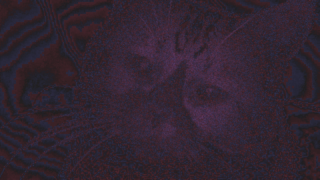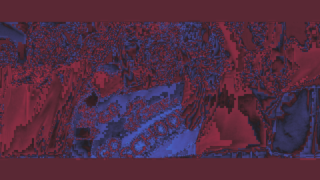## Delta

#### General info

Metric type

full-reference image metric

Value range

(original is much brigther) -1..1 (distorted is much brigther)

Value interpretation

bigger is darker original, 0 - same brightness

MSU VQMT implementations

MSU VQMT visualization

pixel-wise

Available colorspaces

R, G, B, Y, U, V, L

Output values

metric value

Aggregated values

standard set

MSU VQMT usages

-metr delta [over <color components>]

Other names

mean difference

#### Algorithm description

The value of this metric is the mean difference of the color value in the corresponding points of image.

where $m$ – video width, $n$ – video height, image data are in range 0..1. This formula can be rewriten to the following way: $\mbox{mean distorted brightness} − \mbox{mean original brightness}$.

This metric doesn’t show a quality loss, because it can be 0 for completely different images, but you can detect general brightness shifts using this metric if you are sure images have same structure.

#### Benchmark

Choose benchmark:
Implementation System & settings Colors Resolution FPS Sec. per frame
VQMT 14.0 default multithreaded Y HD 720p 557.44 0.003
VQMT 14.0 default multithreaded Y FullHD 1080p 272.49 0.005
VQMT 14.0 default multithreaded Y 4K 2160p 58.9 0.019
VQMT 14.0 default singlethreaded Y HD 720p 179.48 0.007
VQMT 14.0 default singlethreaded Y FullHD 1080p 88.64 0.012
VQMT 14.0 default singlethreaded Y 4K 2160p 22.82 0.046
Measurements were done on VQMT 14.0 BETA r12792 PRO for Windows.
CPU: Intel(R) Core(TM) i7-2600 CPU @ 3.40GHz, 8 cores
GPU: NVIDIA CUDA/GeForce GTX 660 Ti
Values can vary depending on system configuration, input format and other factors
Implementation System & settings Colors Resolution FPS Sec. per frame
VQMT 13.1 default multithreaded Y HD 720p 568.83 0.003
VQMT 13.1 default multithreaded Y FullHD 1080p 260.59 0.005
VQMT 13.1 default multithreaded Y 4K 2160p 54.22 0.02
VQMT 13.1 default singlethreaded Y HD 720p 181.75 0.006
VQMT 13.1 default singlethreaded Y FullHD 1080p 88.77 0.012
VQMT 13.1 default singlethreaded Y 4K 2160p 23.25 0.045
Measurements were done on VQMT 13.1 r12792 PRO for Windows.
CPU: Intel(R) Core(TM) i7-2600 CPU @ 3.40GHz, 8 cores
GPU: NVIDIA CUDA/GeForce GTX 660 Ti
Values can vary depending on system configuration, input format and other factors
Implementation System & settings Colors Resolution FPS Sec. per frame
VQMT 14.0 default multithreaded Y HD 720p 1271.1 0.002
VQMT 14.0 default multithreaded Y FullHD 1080p 646.32 0.004
VQMT 14.0 default multithreaded Y 4K 2160p 119.24 0.016
VQMT 14.0 default singlethreaded Y HD 720p 152.69 0.007
VQMT 14.0 default singlethreaded Y FullHD 1080p 66.5 0.015
VQMT 14.0 default singlethreaded Y 4K 2160p 20.66 0.05
Measurements were done on VQMT 14.0 BETA r12792 PRO for Linux.
CPU: Intel(R) Xeon(R) Silver 4216 CPU @ 2.10GHz, 64 cores
GPU: NVIDIA CUDA/TITAN RTX
Values can vary depending on system configuration, input format and other factors

#### Example

Choose example:OriginalLQ H264, Delta-y=0.0009MSU VQMT visualizationOriginalBlurring, Delta-y=0.0003MSU VQMT visualizationOriginalRandom points, Delta-y=0.0001MSU VQMT visualizationOriginalLuminance shift, Delta-y=0.0078MSU VQMT visualizationOriginalJPEG Q=2, Delta-y=-0.0019MSU VQMT visualizationOriginalJPEG Q=5, Delta-y=-0.0003MSU VQMT visualizationOriginalJPEG Q=10, Delta-y=0.0015MSU VQMT visualizationOriginalJPEG Q=15, Delta-y=-0.0009MSU VQMT visualizationOriginalJPEG Q=20, Delta-y=-0.0002MSU VQMT visualizationOriginalJPEG Q=40, Delta-y=0.0002MSU VQMT visualizationOriginalJPEG Q=80, Delta-y=0.0003MSU VQMT visualization
Choose example:OriginalLQ H264, Delta-y=-0.0093MSU VQMT visualizationOriginalBlurring, Delta-y=0.0046MSU VQMT visualizationOriginalRandom points, Delta-y=0.0042MSU VQMT visualizationOriginalLuminance shift, Delta-y=0.0078MSU VQMT visualizationOriginalJPEG Q=2, Delta-y=0.0047MSU VQMT visualizationOriginalJPEG Q=5, Delta-y=0.0133MSU VQMT visualizationOriginalJPEG Q=10, Delta-y=0.0044MSU VQMT visualizationOriginalJPEG Q=15, Delta-y=0.0063MSU VQMT visualizationOriginalJPEG Q=20, Delta-y=0.0044MSU VQMT visualizationOriginalJPEG Q=40, Delta-y=0.0053MSU VQMT visualizationOriginalJPEG Q=80, Delta-y=0.0042MSU VQMT visualization
Choose example:OriginalLQ H264, Delta-y=0.0072MSU VQMT visualizationOriginalBlurring, Delta-y=0.0001MSU VQMT visualizationOriginalRandom points, Delta-y=0.0001MSU VQMT visualizationOriginalLuminance shift, Delta-y=0.0076MSU VQMT visualizationOriginalJPEG Q=2, Delta-y=-0.0030MSU VQMT visualizationOriginalJPEG Q=5, Delta-y=0.0010MSU VQMT visualizationOriginalJPEG Q=10, Delta-y=-0.0000MSU VQMT visualizationOriginalJPEG Q=15, Delta-y=0.0004MSU VQMT visualizationOriginalJPEG Q=20, Delta-y=0.0001MSU VQMT visualizationOriginalJPEG Q=40, Delta-y=-0.0000MSU VQMT visualizationOriginalJPEG Q=80, Delta-y=0.0000MSU VQMT visualization

#### Legacy notes

Since VQMT 12 metric uses input range 0..1. In legacy mode is assumed input range to be 0..255 and 0..100 for L channel.